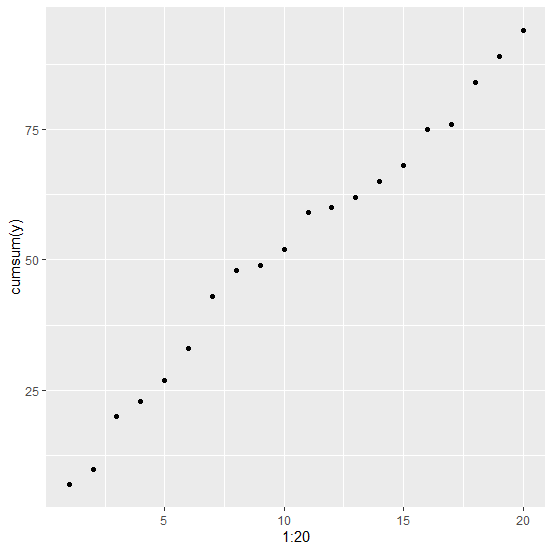# How to create a point chart for cumulative sums using ggplot2 in R?

To create a point chart for cumulative sums using ggplot2, we need to use cumsum function for the dependent variable inside the aes function for aesthetic mapping that describes how the variable will be plotted. For example, if we have a data frame df that contain columns x and y where y is the dependent variable then the point chart for cumulative sums can be created as ggplot(df,aes(1:20,y=cumsum(y)))+geom_point().

## Example

Consider the below data frame −

Live Demo

set.seed(666)
x<-1:20
y<-rpois(20,5)
df<-data.frame(x,y)
df

## Output

  x y
1 1 7
2 2 3
3 3 10
4 4 3
5 5 4
6 6 6
7 7 10
8 8 5
9 9 1
10 10 3
11 11 7
12 12 1
13 13 2
14 14 3
15 15 3
16 16 7
17 17 1
18 18 8
19 19 5
20 20 5

library(ggplot2)
ggplot(df,aes(1:20,y=cumsum(y)))+geom_point()